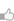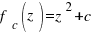求知 文章 文库 Lib 视频 iPerson 课程 认证 咨询 工具 讲座 Modeler Code

 利用python进行数据分析 搭建python环境 统计 1880-2010 年间 全美婴儿姓名的趋势 Ipython & Ipython Notebook NumPy Basics: Arrays and Vectorized Computation Pandas(Python Data Analysis Library) 数据加载、储存与文件格式 绘图和可视化(Matplotlib) 时间序列 经济，金融数据应用 补充例子 国王与囚徒 利用python进行科学计算 分形与混沌之-Mandelbrot集合 分形与混沌之-迭代函数系统(IFS) 分形与混沌之-蔡氏电路模拟 对于μ子实验数据进行快速处理与分析 37%法则 - "非诚勿扰" 中的博弈 关于时间/日期的转换 深入研究 一切从游戏开始:完整的一个 python to hack 实例！ 习题：扑克牌发牌

 415 次浏览4次捐助

Mandelbrot集合

Mandelbrot(曼德布洛特)集合是在复平面上组成分形的点的集合。

Mandelbrot集合的定义(摘自维基百科) Mandelbrot集合可以用下面的复二次多项式定义：# -*- coding: utf-8 -*- # by whyx 2016/6 import numpy as np import pylab as pl from math import log import time from matplotlib import cm def iter_point(c): z = c for i in xrange(1, 100): # 最多迭代100次 if abs(z)>2.0: break # 半径大于2则认为逃逸 z = z*z+c return i # 返回迭代次数 escape_radius = 2.0 iter_num = 100 def smooth_iter_point(c): z = c for i in xrange(1, iter_num): if abs(z)>escape_radius: break z = z*z+c absz = abs(z) if absz > 2.0: mu = i - log(log(abs(z),2),2) else: mu = i return mu # 返回正规化的迭代次数 def draw_mandelbrot(cx, cy, d): # 绘制点(cx, cy)附近正负d的范围的Mandelbrot (x0, x1, y0, y1) = (cx-d, cx+d, cy-d, cy+d) y, x = np.ogrid[y0:y1:200j, x0:x1:200j] c = x + y*1j start = time.clock() mandelbrot = np.frompyfunc(smooth_iter_point,1,1)(c).astype(np.float) print "time=",time.clock() - start #时间单位 秒 pl.imshow(mandelbrot, cmap=cm.Blues_r, extent=[x0,x1,y0,y1]) pl.gca().set_axis_off() x,y = 0.27322626, 0.595153338 pl.subplot(231) draw_mandelbrot(-0.5, 0, 1.5) for i in range(2,7): pl.subplot(230+i) draw_mandelbrot(x, y, 0.2**(i-1)) pl.subplots_adjust(0.02, 0, 0.98, 1, 0.02, 0) pl.show()

 您可以捐助，支持我们的公益事业。 1元 10元 50元 认证码：必填
 415 次浏览4次捐助
 < 首页